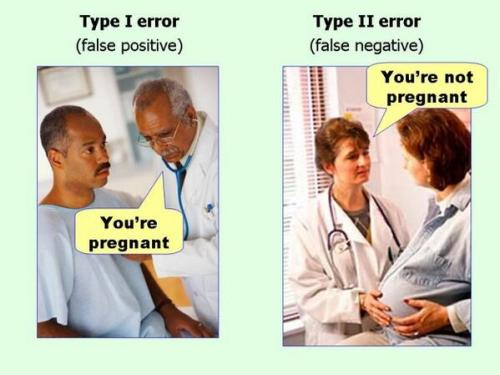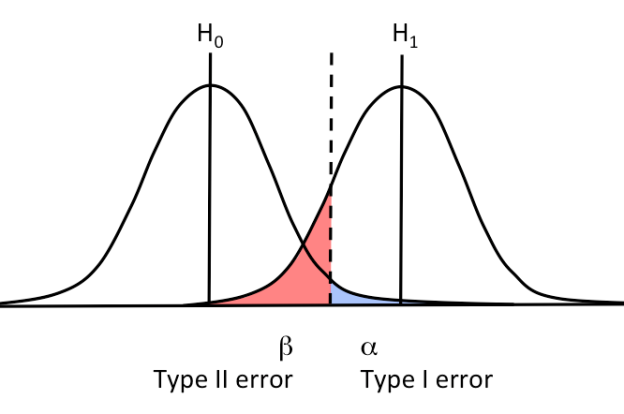# Topic C9 — Hypothesis Testing

## Null hypothesis and alternative hypothesis

• Null hypothesis, $H_0$: presumed default state of nature or status quo. In specifying $H_0$, always contains an equality, i.e. $H_0 =$ or $H_0 \leq$ or $H_0 \geq$.

• Alternative hypothesis, $H_A$: a contradiction of the default state of nature or status quo. Usually, $H_A$ is the outcome we want to test, the “desired” outcome.

We use sample information to infere unknown population parameters. We do hypothesis testing: we determine whether the evidence from the sample contradicts $H_0$.

Outcomes:

• Reject $H_0$: sample evidence inconsistent with $H_0$
• Do not reject $H_0$: evidence not inconsistent with $H_0$

Importantly, we can never accept $H_0$.

• Two-tails: $H_0: \mu = \mu_0$vs $H_A: \mu \neq \mu_0$. If we use $\neq$, we say that both tails matter in order to assess $H_0$. Interpretation of the test: “Has no impact”.
• One-tail: $H_0: \mu \leq \mu_0$vs $H_A: \mu > \mu_0$. Interpretation of the test: “Does not increase”. Look at the sign of $H_A$ to assess which tail to use.

Porcedure

1. Identify the relevant population parameter of interest.
2. Determine whether it is a one or a two-tailed test.
3. Include some form of the equality sign in $H_0$ and use $H_A$ to establish a claim.

## Hypothesis test using CIs

Decision rule

• Reject $H_0$ if the CI does not contain the value of $H_0=\mu_0$
• Do not reject $H_0$ if CI does contain the value of $H_0 = \mu_0$

## Hypothesis test using the p-value

Assume $H_0$ is true and see if sample evidence contradicts it.

The p-value is the likelihood of obtaining a sample statistic at least as extreme as the one in the sample, under the assumption that $H_0$ is true.

If the null hypothesis is true, how likely is that I have a sample at least as extreme as the one I have?

• Caluclate the test statistic of your sample.
• Check what is the area associatied with this $z$
• Check if the are is greater (or smaller, depending on the specification of your test) than the area associated with the significance level you choose.

The p-value tells you the probability that this sample result would be generated if $H_0$ was true. Small p-value = small probability the null is true

## Hypothesis test using the critical value

Reject the null hypothesis if the z-score is greater than some critical value.

• Standardize the sample mean using the sample formula. This is your test statistic.
• Check if it’s greater (or smaller, depending on the type of test) than the $z_{\alpha/2}$ with significance level $\alpha$.

## Test statistics for the poplation mean

Same “brick” of formula that you used to compute CI.

Not in the formula sheet! $\frac{\bar{x}-\mu_0}{\sigma/\sqrt{n}}$ or $\frac{\bar{x}-\mu_0}{s/\sqrt{n-1}}$ if $\sigma$ unknown.

## Test statistics for the population proportion

Not in the formula sheet! $\frac{\bar{p}-p_0}{\sqrt{\frac{p_0(1-p_0)}{n}}}$

## Type I and Type II errors

• Type I: $H_0$ is true but we believe in $H_A$ (i.e. we don’t believe in $H_0$).
• Type II: $H_0$ is not true but we believe in $H_0$ (i.e. we don’t believe in $H_A$).

• Type I: we reject $H_0$ when $H_0$ is true.
• Type II: we fail to reject $H_0$ when $H_0$ is false.

• Type I: measured by $\alpha$, significance level
• Type II: measured by $\beta$, power of the test.

Both depend of the standard error.

$H_0$ true $H_0$ not true
Reject $H_0$ Type I error
False positive
$\alpha$
Correct decision
Power
$1-\beta$
Do not reject $H_0$ Correct decision
Confidence
$1-\alpha$
Type II error
False negative
$\beta$

A decrease in $\alpha$ will increase $\beta$.

If the null hypothesis is “not pregnant”, the following show type I and II error.